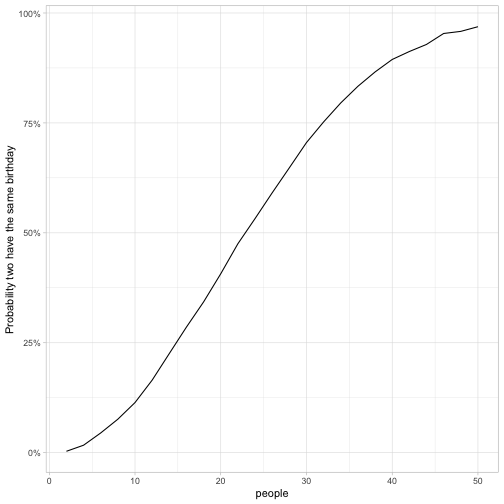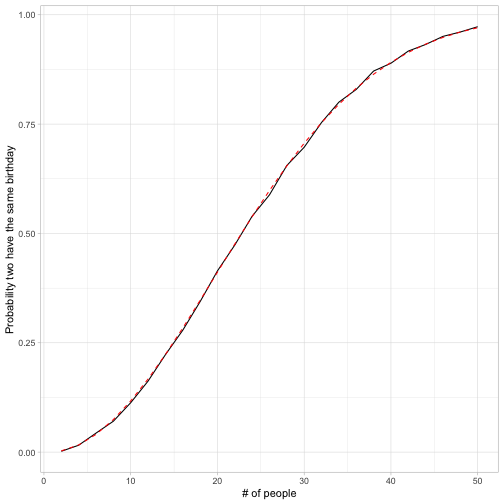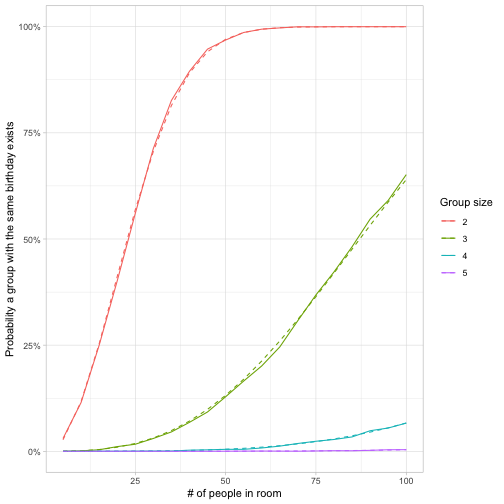### David Robinson

Principal Data Scientist at Heap, works in R and Python.

# The birthday paradox puzzle: tidy simulation in R

Previously in this series:

The birthday problem is a classic probability puzzle, stated something like this.

A room has n people, and each has an equal chance of being born on any of the 365 days of the year. (For simplicity, we’ll ignore leap years). What is the probability that two people in the room have the same birthday?

If you’re not familiar with the puzzle, you might expect that in a moderately sized room (like 20-30 people) that the chance will be pretty small that two people have the same birthday. But in fact, if the room has more than 23 people, the chance is greater than 50%! This makes the puzzle a classic for intro statistics classes.

I’ve been interested for a while in the tidyverse approach to simulation. In this post, I’ll use the birthday problem as an example of this kind of tidy simulation, most notably the use of the underrated crossing() function.

First, I’ll show the combined approach, before breaking it down.This visualization shows that the probability two people have the same birthday is low if there are 10 people in the room, moderate if there are 10-40 people in the room, and very high if there are more than 40. It crosses over to become more likely than not when there are ~23 people in the room.

I’ll break down the simulation a bit below.

#### Simulating one case

When you’re approaching a simulation problem, it can be worth simulating a single case first.

Suppose we have 20 people in a room. Ignoring leap years (and treating each calendar day as a number from 1 to 365), we can simulate their birthdays with sample(365, 20, replace = TRUE).

We then use two handy base R functions, duplicated and any, to discover if there are any duplicated days.

This gives us a one-liner for simulating a single case of the birthday problem, with (say) 20 people:

If you run this line a few times, you’ll see it’s sometimes true and sometime false, which already gives us the sense that pairs of people sharing a birthday in a moderately-sized room aren’t as rare as you might expect.

#### The tidy approach to many simulations across multiple parameters

I find the crossing() function in tidyr incredibly valuable for simulation. crossing() creates a tibble of all combinations of its arguments.

Our simulation starts by generating 10000 * 25 combinations of people and trial, with values of people ranging from 2 to 50. trial exists only so that we have many observations of each, in order to minimize statistical noise.

We then use functions from purrr, useful for operating across a list, to generate a list column of integer vectors. With a second step, we determine which of them have multiples of any birthday.

Finally, we use one of my favorite tricks in R: using mean() to find the fraction of a logical vector that’s TRUE.

Together these three steps (crossed+sim+summarized) make up the solution in the first code chunk above. Notice this simulation combines the base R approach (sample, duplicated, and any) with tidyverse functions from dplyr (like mutate, group_by, and summarize), tidyr (crossing) and purrr (map and map_lgl). I’ve found this crossing/map/summarize approach useful in many simulations.

How can we check our math? R has a built-in pbirthday function that gives an exact solution: for instance, pbirthday(20) gives the probability two people in a room of 20 will have the same birthday. We can compare our suLooks like we got very close!

### Generalizing the birthday problem

We’ve seen how likely it is that there’s a pair of people with the same birthday. What about a group of 3, 4, or 5 who all have the same birthday? How likely is that?

First, instead of working with ~ any(duplicated(.)) as our vectorized operation, we could find how many people are in the most common group of birthdays, with another combination of base R functions: max and table.

Now that we know within each trial what the largest group with the same birthday is. We now need to know the fraction within each number where the most_common is at least 2, 3, 4, or 5. We use another application of the ever-useful crossing function, to apply multiple thresholds to each number of people.

We can visualize these and compare them to the exact values from pbirthday() (pbirthday() takes a coincident argument to extend the problem to more than two participants).From this we learn that in a room of 100 people, it is almost certain that a pair exists with the same birthday, more likely than not that that a group of 3 does, unlikely that a group of 4 does, and almost impossible that a group of 5 does.

What I like about the tidy approach to simulation is that we can keep adding parameters that we’d like to vary through an extra crossing() and an extra term in the group_by. For instance, if we’d wanted to see how the probability varied as the number of days in a year changed (not relevant for birthdays, but might be relevant in other applications like the cryptographic birthday attack), we could have added that to the crossing() and worked with the first argument to sample.